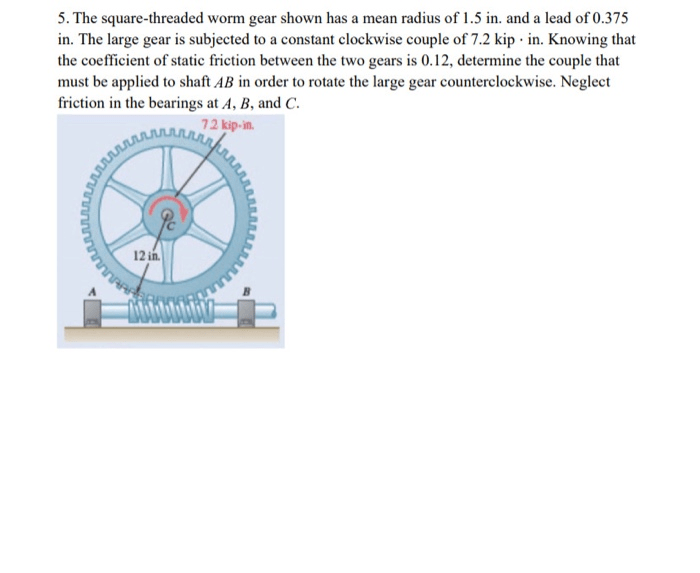# The square-threaded worm gear shown has a mean radius of 1.5 in. and a lead of 0.375 in. The large gear is subjected to a constant clockwise couple of 7.2 kip·in. Knowing that the coefficient of static friction between the two gears is 0.12, determine the minimum couple that must be applied to shaft AB in order to rotate the large gear counterclockwise. Neglect friction in the bearings at A, B, and C.

Question-AnswerCategory: Engineering MechanicsThe square-threaded worm gear shown has a mean radius of 1.5 in. and a lead of 0.375 in. The large gear is subjected to a constant clockwise couple of 7.2 kip·in. Knowing that the coefficient of static friction between the two gears is 0.12, determine the minimum couple that must be applied to shaft AB in order to rotate the large gear counterclockwise. Neglect friction in the bearings at A, B, and C.

The square-threaded worm gear shown has a mean radius of 1.5 in. and a lead of 0.375 in. The large gear is subjected to a constant clockwise couple of 7.2 kip·in. Knowing that the coefficient of static friction between the two gears is 0.12, determine the minimum couple that must be applied to shaft AB in order to rotate the large gear counterclockwise. Neglect friction in the bearings at A, B, and C.